# Aptitude - Compound Interest

1.
A bank offers 5% compound interest calculated on half-yearly basis. A customer deposits Rs. 1600 each on 1st January and 1st July of a year. At the end of the year, the amount he would have gained by way of interest is:
Rs. 120
Rs. 121
Rs. 122
Rs. 123
Explanation:
Amount
 = Rs.1600 x1 + 52 + 1600 x1 + 52 x 100 2 x 100
 = Rs.1600 x 41 x 41 + 1600 x 4140 40 40
 = Rs.1600 x 4141 + 140 40
 = Rs.1600 x 41 x 8140 x 40
= Rs. 3321.C.I. = Rs. (3321 - 3200) = Rs. 121

2.
The difference between simple and compound interests compounded annually on a certain sum of money for 2 years at 4% per annum is Re. 1. The sum (in Rs.) is:
625
630
640
650
Explanation:

Let the sum be Rs. x. Then,

 C.I. =x1 + 42 - x=676 x - x= 51 x. 100 625 625

 S.I. =x x 4 x 2= 2x . 100 2551x - 2x = 1 625 25x = 625.

3.
There is 60% increase in an amount in 6 years at simple interest. What will be the compound interest of Rs. 12,000 after 3 years at the same rate?
Rs. 2160
Rs. 3120
Rs. 3972
Rs. 6240
None of these
Explanation:

Let P = Rs. 100. Then, S.I. Rs. 60 and T = 6 years.R =100 x 60= 10% p.a. 100 x 6

Now, P = Rs. 12000. T = 3 years and R = 10% p.a.C.I.
 = Rs.12000 x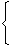1 + 103 - 1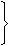100
 = Rs.12000 x 3311000
= 3972.

4.
What is the difference between the compound interests on Rs. 5000 for 1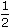years at 4% per annum compounded yearly and half-yearly?
Rs. 2.04
Rs. 3.06
Rs. 4.80
Rs. 8.30
Explanation:

C.I. when interest
compounded yearly
 = Rs.5000 x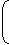1 + 4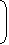x1 +x 4100 100
 = Rs.5000 x 26 x 5125 50
= Rs. 5304.

C.I. when interest is
compounded half-yearly
 = Rs.5000 x1 + 23100
 = Rs.5000 x 51 x 51 x 5150 50 50
= Rs. 5306.04Difference = Rs. (5306.04 - 5304) = Rs. 2.04

5.
The compound interest on Rs. 30,000 at 7% per annum is Rs. 4347. The period (in years) is:
2
 2 1 2
3
4
 Then, 300001 + 7n = 34347 100107n = 34347 = 11449 =1072 100 30000 10000 100n = 2 years.